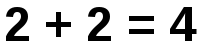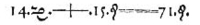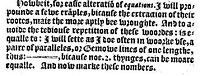# Equals sign

﻿
Equals signA well-known equality featuring the equals sign, often used in the phrases, "put two and two together," and, "As easy as two plus two."

The equality sign, equals sign, or "=" is a mathematical symbol used to indicate equality. It was invented in 1557 by Robert Recorde. The equals sign is placed between the things stated to have the same value, as in an equation. It is the Unicode and ASCII character 003D (in hexadecimal).

## History

The etymology of the word equal is from the Latin word aequalis, meaning "uniform, identical, or equal," from aequus "level, even, just."The first use of an equals sign, equivalent to 14x+15=71 in modern notation. From The Whetstone of Witte by Robert Recorde.Recorde's introduction of "="

The "=" symbol that is now universally accepted by mathematics for equality was first recorded by Welsh mathematician Robert Recorde in The Whetstone of Witte (1557). The original form of the symbol was much wider than the present form. In his book Recorde explains his design of the "Gemowe lines" (meaning twin lines, from the Latin gemini):

...to auoide the tediouſe repetition of theſe woordes : is equalle to : I will ſette as I doe often in woorke vſe, a paire of paralleles, or Gemowe lines of one lengthe, thus: =, bicauſe noe .2. thynges, can be moare equalle.

...to avoid the tedious repetition of these words: "is equal to", I will set (as I do often in work use) a pair of parallels (or Gemowe lines) of one length (thus =), because no two things can be more equal.

According to Scotland's St Andrews University Maths History website:

The symbol '=' was not immediately popular. The symbol || was used by some and æ (or œ), from the Latin word aequalis meaning equal, was widely used into the 1700s.

## Usage

In mathematics, the equals sign is used for definitions (ex: let x = 2) as well as conditions and denoted equalities (ex: if x = 2 then x/2 = 1).

The equals sign is also used as a grammatical tone letter in the orthographies of Budu in the Congo-Kinshasa, in Krumen, Mwan and Dan in the Ivory Coast. The Unicode character used for the tone letter (U+A78A) is different from the mathematical symbol (U+003D).

### In computer programming

Traditional computer programming languages and their descendants (e.g. the Algol family, Pascal family, ADA, Eiffel, etc) use the equals sign as a boolean equality-operator as well as for defining constants, types, and parameters. This is consistent with mathematical usage and marks an intention for such languages to be as similar as possible to mathematical notation. A colon-equals (`:=`) is typically used to denote assignment.

In C-like languages, it is instead used as an assignment operator. C-like languages therefore use a double equals sign (`==`) as an equality operator.

Some languages (e.g. BASIC) use a single equals sign to denote both functions, sometimes with an optional `let` keyword to resolve any ambiguities.

#### Usage of several equal-signs

In PHP, the triple equals sign (`===`) denotes identicalness, meaning that not only do the two values evaluate to the same boolean value, they are also of the same data type. For instance, the expression `0 == false` is true, but `0 === false` is not, because the number 0 is an integer value (whereas false is a boolean).

JavaScript has the same semantics for `===`, referred to as "equality without type coercion". However in JavaScript the behavior of `==` cannot be described by any simple consistent rules. The expression `0 == false` is true, but `0 == undefined` is false, even though both sides of the `==` act the same in boolean context. For this reason it is recommended to avoid the `==` operator in JavaScript in favor of `===`.

In Ruby, equality under `==` requires both operands to be of identical type, e.g. `0 == false` is false. The `===` operator is flexible and may be defined arbitrarily by any given type. For example a value of type `Range` is a range of integers, such as `1800..1899`. `(1800..1899) == 1844` is false, since the types are different (Range vs. Integer); however `(1800..1899) === 1844` is true, since Range defines `===` to mean "inclusion in the range". Note that under these semantics, `===` is non-commutative; e.g. `1844 === (1800..1899)` is false, since it is interpreted to mean `Integer#===` rather than `Range#===`.

## Related symbols

### Approximately equal

Symbols used to denote items that are approximately equal are "wavy" equals signs.

• (Unicode 2248),
• (Unicode 2243), a combination of ≈ and =, also used to indicate asymptotically equal to
• (Unicode 2245), another combination of ≈ and =, which is also sometimes used to indicate isomorphism or congruence
• ~ (Unicode 007E), which is also sometimes used to indicate proportionality
• (Unicode 2252), in Japanese, this is commonly used.

### Not equal

The symbol used to denote inequation — when items are not equal — is a slashed equals sign "≠" (Unicode 2260).

Most programming languages, limiting themselves to the ASCII character set, use `~=`, `!=`, `/=`, `=/=`, or `<>` to represent their boolean inequality operator.

### Identity

The triple bar symbol "≡" (U+2261) is often used to indicate an identity, a definition (which can also be represented by "≝", U+225D), or a congruence relation in modular arithmetic. The symbol "" can be used to express that an item corresponds to another.

### Isomorphism

The symbol "" is often used to indicate isomorphic algebraic structures or congruent geometric figures.

### In logic

Equality of truth values, i.e. bi-implication or logical equivalence, may be denoted by various symbols including =, ~, and ⇔.

### In a double-barreled name

A possibly unique case of the equal sign in a person's name, specifically in a double-barreled name, was by pioneer aviator Alberto Santos=Dumont, as he is also known to not only have often used an equal sign (=) between his two surnames in place of a hyphen, but also seems to have personally preferred that practice, to display equal respect for his father's French ethnicity and the Brazilian ethnicity of his mother.

## Misconceptions

The equals sign can be used incorrectly within a mathematical argument, if used in a manner that connects steps of math in a non-standard way, rather than to show equality. For example, if one were finding the sum of the numbers 1, 2, 3, 4, and 5, one might say: 1 + 2 = 3 + 3 = 6 + 4 = 10 + 5 = 15. This is clearly incorrect, because each part of the equality has a different value. If interpreted strictly as it says, it implies 3 = 6 = 10 = 15 = 15. A correct version of the argument would be 1 + 2 = 3, 3 + 3 = 6, 6 + 4 = 10, 10 + 5 = 15.

Wikimedia Foundation. 2010.

### Look at other dictionaries:

• equals sign — BrE equal sign AmE n the sign (=) that you use in mathematics to show that two things are the same size, number, or amount …   Dictionary of contemporary English

• equals sign — (also equal sign) ► NOUN ▪ the symbol …   English terms dictionary

• equals sign — noun see equal sign * * * ˈequals sign 7 [equals sign] (also ˈequal sign) noun the symbol (=), used in mathematics • …   Useful english dictionary

• equals sign — UK / US noun [countable] Word forms equals sign : singular equals sign plural equals signs maths the sign = used to show that two sets of numbers, letters, or symbols are the same in quantity or amount …   English dictionary

• equals sign — BrE equal sign AmE noun (C) a sign used in mathematics to show that two things are the same size, number, or amount: In the equation x=y, x is to the left of the equals sign …   Longman dictionary of contemporary English

• equals sign — lygybės ženklas statusas T sritis automatika atitikmenys: angl. equals sign vok. Gleichheitszeichen, n rus. знак равенства, m pranc. signe égal, m …   Automatikos terminų žodynas

• equals sign — noun see equal sign …   New Collegiate Dictionary

• equals sign — (also equal sign) noun the symbol …   English new terms dictionary

• ˈequals ˌsign — noun [C] the sign = used in mathematics to show that two sets of numbers are the same in quantity or amount …   Dictionary for writing and speaking English

• equals sign — /ˈikwəlz saɪn/ (say eekwuhlz suyn) noun the symbol =, used to show that two things are equal. Also, equal sign …   Australian English dictionary